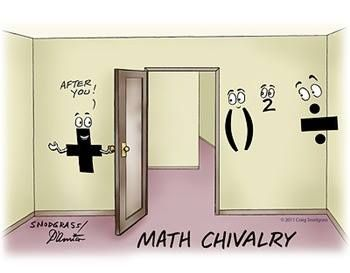Evaluating Expressions
3 years ago
kharalambopoulos
Save
Edit
Host a game
Live GameLive
Homework
Solo Practice
Practice11 QuestionsShow answers
• Question 1
180 seconds
Q. What is the value of the expression
x2 + y2 + x - y
when x = -1 and y = 3
6
8
12
16
• Question 2
180 seconds
Q. evaluate bc + 5a
when  a = 3, b = 4, and c = -6
9
38
-38
-9
• Question 3
180 seconds
Q. evaluate bc + 5a
when  a = 3, b = 4, and c = -6
9
38
-38
-9
• Question 4
180 seconds
Q. a2-b2Evaluate if a=4 and b =-2
4
20
-4
12
• Question 5
300 seconds
Q. g2-3(h-g)Evaluate if g=3 and h=-2
6
9
24
5
• Question 6
180 seconds
Q. What is the first step in solving this problem:
-12 ÷ 6 (-3) + 8
multiplication
division
subtraction
• Question 7
300 seconds
Q. Solve the following:
-12 ÷ 6 (-3) + 8
-14
2
-22
3
• Question 8
180 seconds
Q. Solve the following:
8 + (-4) ÷ 4
-7
1
-1
7
• Question 9
180 seconds
Q. Solve the following:
-5 (- 6) + 10
-1
40
-20
21
• Question 10
180 seconds
Q. Solve the following:
3 + 27 ÷ (-3)
-6
-10
10
-12
• Question 11
180 seconds
Q. Solve the following:
-14 ÷ 2 – 15